﻿ Digital Circuits and Systems - Circuits i Sistemes Digitals (CSD) - EETAC - UPCBachelor's Degree in Telecommunications Systems and in Network Engineering## Using WolframAlpha to analyse simple circuits

Boolean algebra calculator (analysis method II)

1. Specifications

The aim is to obtain a circuit's truth table directly from its boolean algebraic equation using the calculator from For instance, the circuit represented in Fig. 1.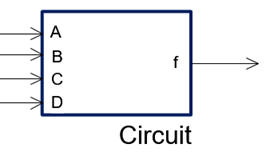f = g(A, B, C, D) Fig. 1. Circuit to be analysed.

2. Plan

As shown in Fig. 2, the circuit equation is the same concept as the circuit logic diagram.Fig. 1. Circuit to be analysed and its equation (visio).

A. Analyse the circuit network to deduce the equation.

B. Translate the equation to WolframAlpha language, step by step saving partial results in a text file. Start with a simple factor and run the engine to check results. Repeat until the equation is completed. This is where to place the text file with your equations and other documents or pictures:

C:\CSD\P1\Wolfram\ (files)

C. Examine the order of the truth table input variables and rearrange them if necessary to obtain the final truth table.

D. Check solutions with other team mates or with results from other analysis methods.

E. Produce an original handwritten report following the usual template and including pictures, diagrams, computer results and pen annotations and comments.

3. Development

A) The circuit and its algebraic equivalent equation

f = g(A, B, C, D)= ( (A + B)·C’·(A·D)’ )’

B) Write the equations.txt into WolframAlpha and run the software to obtain the truth table:NOTE: Perhaps, sometimes, if the logic equation is too large, it may not be correctly understood by WolframAlpha. In such cases, it may be convenien to simplify input notation. For instance:

G = f(D1, D0, A, B) = f(R, S, A, B)

C) This is an example of computer results that must be interpreted. In this case, the input columns have to be rearranged in order to deduce the correct terms.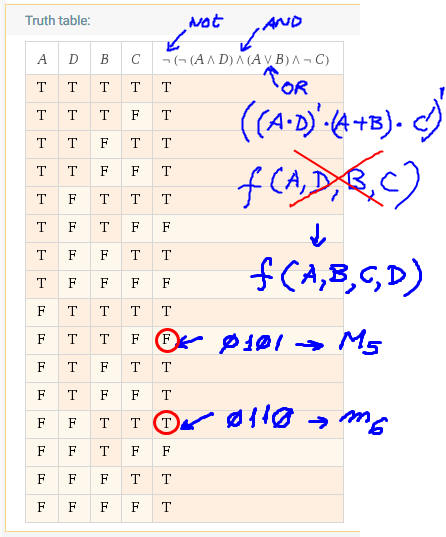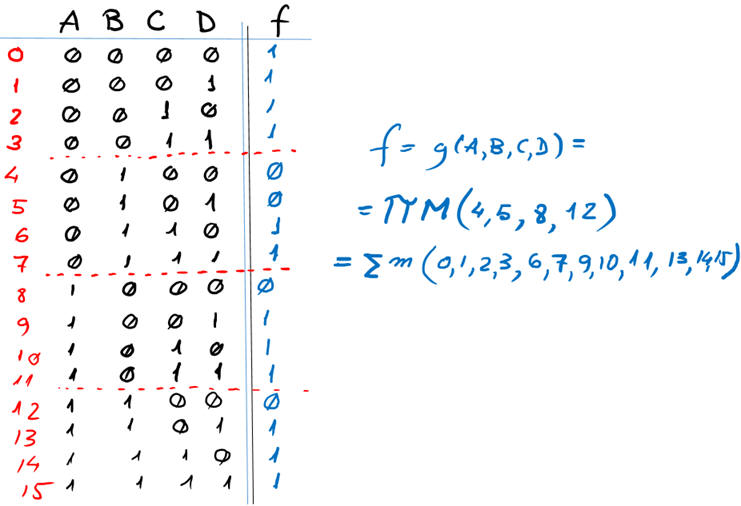Additionally, you may like to obtain the circuit representation: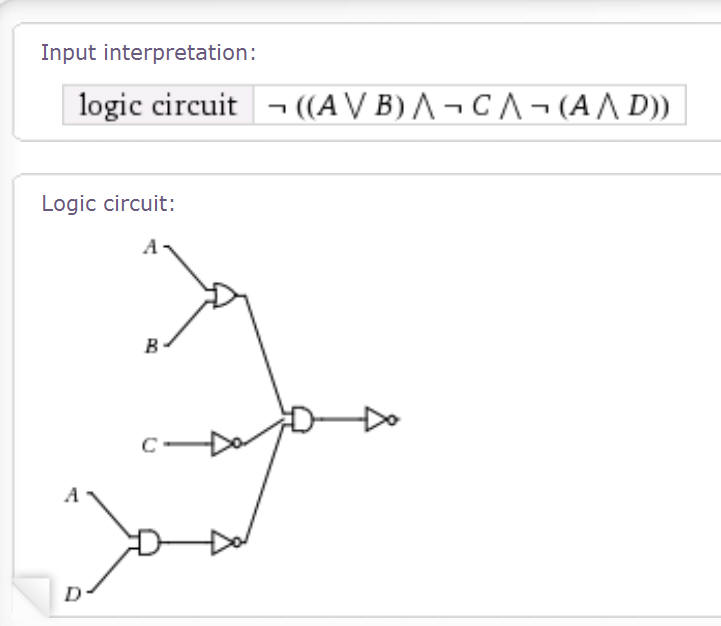Other applications

And sure, you can use WolframAlpha to calculate anything you like, for instance, functions and derivatives:y(x) = 5sin^2(x^4)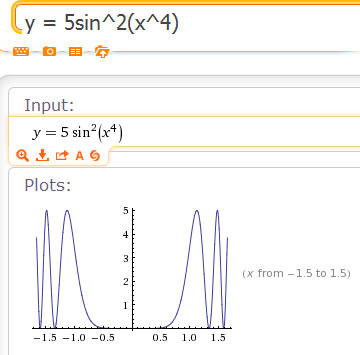The first derivative y'(x) = dy/dx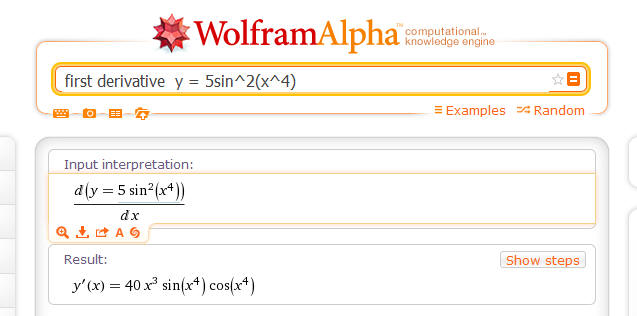And for instance:  integrate ( sqr (4-x^2) (x^3 cos(x/2) + 1/2 ) )dx from -2 to 2